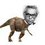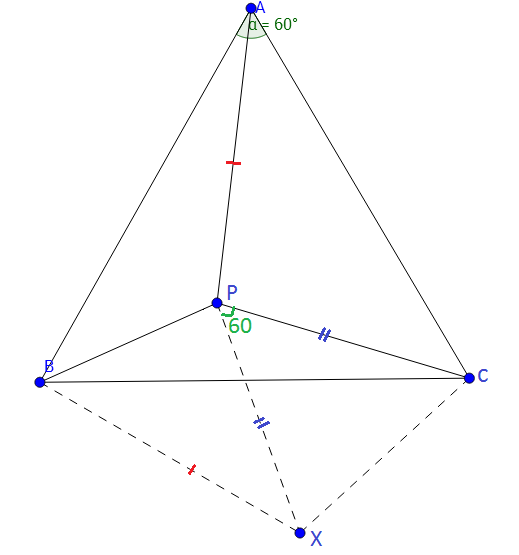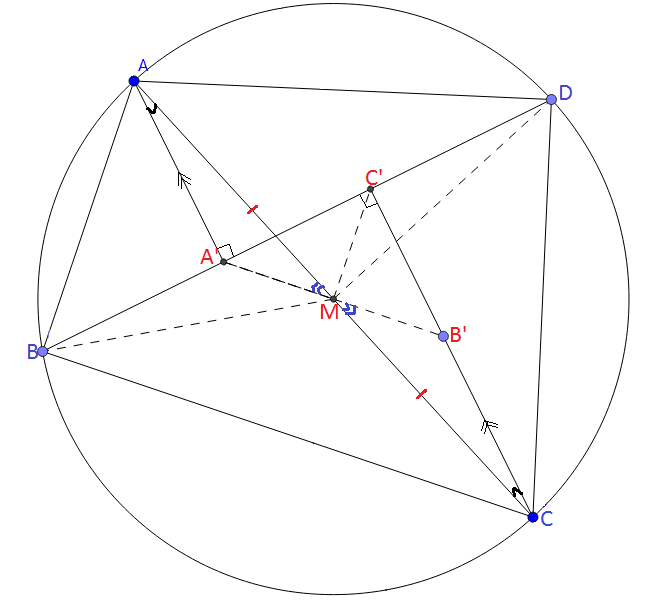# RMO Board Part 2

Since the previous board was overcrowded with comments , I decided to create a new note so as to keep this step lively. If this also gets crowded , eventually we will again have new RMO board.What you have to do:

1) Propose a problem in the comments. Then I will add that problem here in this note content by giving the respective credit. And after I add the problem , the comment of proposing the problem must be deleted by the problem poster.

2) The problem poster must not post solution to his/her own problem unless someone from our community posts or no one gets it right even after considerable period of time.

3) Inappropriate / trivial comments are not allowed and must be deleted if posted by chance.An enthusiastic discussion is expected.

5)The problems to which solutions are posted will be accompanied by a checkmark $\large(\checkmark)$ at the end.

Problems:

Q1) Find all prime numbers $p$ for which there are integers $x,y$ satisfying $p+1=2x^2$ and $p^2+1=2y^2$.$\large\checkmark$

Q2) The roots of the equation $x^3-3ax^2+bx+18c=0$ form a non-constant arithmetic progression and the roots of the equation $x^3+bx^2+x-c^3=0$ form a non-constant geometric progression. Given that $a,b,c$ are real numbers, find all positive integral values $a$ and $b$.(Shared by Mycobacterium Tuberculae) $\large\checkmark$

Q3) In an equilateral $\Delta ABC$ , $P$ is a point inside the triangle such that $PA^2=PB^2+PC^2$.Prove that $m\angle BPC = 150^\circ$.(Shared by Raven Herd) $\large\checkmark$

Q4) Evaluate $\large\binom{n}{0} + \binom{n}{3} + \binom{n}{6} + \binom{n}{9} + ... \binom{n}{n}$ with respect to $n$. (Shared by Svatejas) $\large\checkmark$

Q5) Consider a cyclic quadrilateral $ABCD$ such that $AC$ is the diameter of its circumcircle. Construct points $A'$ and $C'$ on $BD$ such that $AA' \perp BD$ and $CC' \perp BD$ . Show that $DA'=C'B$.(Shared by Karthik V)

Q6) Prove that polynomial $az^n+z+1$ has at least one root in $|z|\leq 2$. (Shared by Shivamani P)

Q7) If $a,b,c$ are positive reals , determine the minimum value of the expression $ab(a-c)+bc(b-a)+ca(c-b)$ with proof. (Posed by Nihar M) $\large\checkmark$

Q8)a) How many ways are there to represent a natural number $n$ as a sum of $k$ natural numbers?

Q8)b) How many ways are there to represent a natural number $n$ as a sum of $k$ non-negative numbers?

Q9) Find all ordered pairs of integers $(m,n)$ satisfying: $3\times 2^m + 1= n^2$.

Q10)a) Prove that if $x^2+px-q$ and $x^2-px+q$ both factorize into linear factors with integral coefficients, then the positive integers and are respectively the hypotenuse and area of a right angled triangle sides of integer lengths.

Q10)b) Show further that if $x^2+px-q = (x-\alpha)(x-\beta) \ and \ x^2-px+q = (x-\gamma)(x-\delta)$ where $p,q,\alpha,\beta,\gamma,\delta$ are integers, $\alpha,\beta,\gamma,\delta$ then are numerically the radii of the incircle and the three excircles of the triangle. (Shared by Svatejas S)Note by Nihar Mahajan
5 years, 1 month ago

This discussion board is a place to discuss our Daily Challenges and the math and science related to those challenges. Explanations are more than just a solution — they should explain the steps and thinking strategies that you used to obtain the solution. Comments should further the discussion of math and science.

When posting on Brilliant:

• Use the emojis to react to an explanation, whether you're congratulating a job well done , or just really confused .
• Ask specific questions about the challenge or the steps in somebody's explanation. Well-posed questions can add a lot to the discussion, but posting "I don't understand!" doesn't help anyone.
• Try to contribute something new to the discussion, whether it is an extension, generalization or other idea related to the challenge.

MarkdownAppears as
*italics* or _italics_ italics
**bold** or __bold__ bold
- bulleted- list
• bulleted
• list
1. numbered2. list
1. numbered
2. list
Note: you must add a full line of space before and after lists for them to show up correctly
paragraph 1paragraph 2

paragraph 1

paragraph 2

[example link](https://brilliant.org)example link
> This is a quote
This is a quote
    # I indented these lines
# 4 spaces, and now they show
# up as a code block.

print "hello world"
# I indented these lines
# 4 spaces, and now they show
# up as a code block.

print "hello world"
MathAppears as
Remember to wrap math in $$ ... $$ or $ ... $ to ensure proper formatting.
2 \times 3 $2 \times 3$
2^{34} $2^{34}$
a_{i-1} $a_{i-1}$
\frac{2}{3} $\frac{2}{3}$
\sqrt{2} $\sqrt{2}$
\sum_{i=1}^3 $\sum_{i=1}^3$
\sin \theta $\sin \theta$
\boxed{123} $\boxed{123}$

Sort by:

Q4) Let $f(x) = (1+x)^{n} ={n \choose 0}+ {n \choose 1} x + \ldots + {n \choose n} x^{n}$

Now, $f(1) + f(\omega) +f(\omega ^2) = {n \choose 0} (1+ \omega + \omega ^2) + {n \choose 1} (1+ \omega ^{2} + \omega ^{4}) + \ldots + {n \choose n} (1+ \omega ^n + \omega ^2n)$ $= 3 \left( {n \choose 0} + {n \choose 3}+ {n \choose 6} + \ldots \right)$

So, ${n \choose 0} + {n \choose 3}+ {n \choose 6} + \ldots = \dfrac{f(1) + f(\omega) +f(\omega ^2)}{3} = \dfrac{2^n + (- \omega ^2)^n + ( - \omega)^n}{3}$.

So, we can manipulate the value of this expression by knowing the value of $n$.

- 5 years, 1 month ago

@Surya Prakash Great job!! Can you simply this further to eliminate $\omega$?

- 5 years, 1 month ago

Q1) It is clear that $p(p-1) = 2(y+x)(y-x)$. If $p|y-x$ it implies that $p \leq y-x$, then it should imply that $p-1 \geq 2(y+x)$. But it is not possible. So, $p$ does not divide $y-x$. So, $p|y+x$ it implies that $p \leq y+x$, but this implies that $p-1 \geq 2(y-x)$. So, we get $p +1 \leq 4x$. Which reduces to $2x^2 \leq 4x$, $x \leq 2$. If $x=1$, then $p=1$, a contradiction. And $x=2$ implies that $p=7$. Therefore, $p=\boxed{7}$ is the only solution.

- 5 years, 1 month ago

For the $2nd$ question,

Let the roots of first equation be $m-d,m,m+d$

Therefore we get that, $m-d+m+m+d=3a=3m$. Hence $m=a$

Then by applying Vieta's formula for other two, we get that, $3{m}^{2}-{d}^{2}=b$ and ${m}^{3}-m{d}^{2}=-18c$

Let the roots for the second equation be $n/r,n,nr$

Therefore by applying Vieta's fomula,we get that, $n=c$ and $n(1/r+1+r)=-b$ and ${n}^{2}(1/r+1+r)=1$

From here we get that, $n=-1/b=c$

Substituting these values in the previously acquired equations we get that, ${m}^{3}-m(3{m}^{2}-b)=18/b$

Simplifying we get that, $a{b}^{2}-2{a}^{3}b-18=0$

Applying formula for quadratic roots, $b=\frac{{a}^{3}\pm \sqrt{{a}^{6}+18a}}{a}$

For $b$ to be an integer, ${a}^{6}+18a={k}^{2}$

We see that, ${a}^{3}

From here we see that, $a=2$ only satisfies. Therefore the required solutions are $a=2$ and $b=9$.

- 5 years, 1 month ago

My solution for the $3rd$ one is quite big. I will just give some hints to solve it.

1. Try it by co-ordinate geometry.

2. Take $B$ as $(-1/2,0)$ and $C$ as $(1/2,0)$.Therefore,we get $A$ as $(\sqrt{3}/2,0)$.

3. Then take $P$ as$(x,y)$ use the provided equation of ${PA}^{2}={PB}^{2}+{PC}^{2}$ to get the equation of locus of $P$.The equation comes out to be ${x}^{2}+{y}^{2}+\sqrt{3}y-1/4=0$

4. Then take the $X-Y$ plane as the Argand plane and apply the Rotation theorem of Complex numbers for $B,P,C$ in order.Here the complex numbers are, i. $B=-1/2$, ii. $C=1/2$ and iii. $P=x+iy$

5. By simplifying the equation obtained in the $4th$ step, we get that $\angle{BPC}={150}^{\circ}$

You may consider this as a solution because I have provided all the steps required.(But this step completely depends on you :P )

- 5 years, 1 month ago

$Nice one:$ But Here is a still simpler one :)

Solution to $3rd$ stuff$Construction$: Draw $PX$ = $PC$ such that $\angle CPX = 60^{\circ}$. Finally join $BX$ and $CX$.

Clearly $\Delta CPX$ is equilateral. => $CX$ = $PC$ ------ $1$ and Also, $\angle PCX = \angle ACB$ = $60^{\circ}$ => $\angle PCX - \angle PCB = \angle ACB - \angle PCB$ => $\angle BCX = \angle ACP$ ------- $2$

From the results in $eq^{n}$ $1$, $2$ and that $BC$ = $AC$, we conclude that $\Delta BCX \cong \Delta ACP$. => $BX$ = $PA$

But $Given$ $PB^{2} + PC^{2}$ = $PA^{2}$

=> $PB^{2} + PX^{2}$ = $BX^{2}$ ............... [$PC$ = $PX$ and $BX = PA$]

Therefore by the converse of $Pythagoras... theorem$, it is obvious that $\angle BPX$ = $90^{\circ}$

Thus the required angle = $\angle BPC$ = $\angle BPX + \angle CPX$ = $90^{\circ}$ + $60^{\circ}$ = $150^{\circ}$.

- 3 years, 7 months ago

1) p = 7

$p(p - 1) = 2(y + x)(y - x)$

comparing of y + x and p gives p = 7

- 5 years, 1 month ago

Sorry to say , but you have dropped out some cases. This solution is incomplete.

- 5 years, 1 month ago

let me check

p can't divide 2 and y - x so p divides only y + x and by some observations, i found x is equal to or smaller than 2, so when x = 1 then p = 1. No. then x = 2 so p = 7

- 5 years, 1 month ago

- 5 years, 1 month ago

@Dev Sharma Kindly always post a full solution.

- 5 years, 1 month ago

7) @Nihar Mahajan We have $ab(a-c)+bc(b-a)+ca(c-b)$.

Multiplying we get $a^2b+b^2c+c^2a-3abc= abc(\frac {a}{c}+ \frac {b}{a} + \frac {c}{b}-3$).

By AM-GM inequality, $\frac {a}{c}+ \frac {b}{a} + \frac {c}{b} \ge 3$.

Therefore, minimum value of the expression $\frac {a}{c}+ \frac {b}{a} + \frac {c}{b}$ is $3$.

Therefore, minimum value of $ab(a-c)+bc(b-a)+ca(c-b)$ is $0$ and equality occurs when $a=b=c=0$.

- 5 years, 1 month ago

@Surya Prakash @Nihar Mahajan @Svatejas Shivakumar There seems to be a blunder in problem statement of Q4). I guess it meant " Simplify $\large \binom{n}{0} + \binom{n}{3} + \binom{n}{6} + \binom{n}{9} + ... \binom{n}{ 3 \cdot \lfloor \frac{n}{3} \rfloor }$." The last summand being $\binom{n}{n}$ doesn't make sense at all if $n$ is not a multiple of $3$.

- 5 years, 1 month ago

Its correct.

- 5 years, 1 month ago

Try substituting $n = 4$ in the summation. The link between last term of the summation ( i.e. last summand ) and previous summands is not clear.

- 5 years, 1 month ago

It satisfies.

- 5 years, 1 month ago

Let $f(n) = \large\binom{n}{0} + \binom{n}{3} + \binom{n}{6} + \binom{n}{9} + ... \binom{n}{n}$.Then $f(4) = \binom{4}{0} + \binom{4}{3} + \binom{4}{4}$ ? I am certainly sure about the last summand being $\binom{n}{ 3 \cdot \lfloor \frac{n}{3} \rfloor }$, contrary to $\binom{n}{n}$ mentioned in the question.

- 5 years, 1 month ago

In that way!!!!! Then it is understood that it should be $3.[\frac{n}{3}]$

- 5 years, 1 month ago

I guess it would have been understood if it was just ..... , but rather it mentioned the last summand wrong 😓.

- 5 years, 1 month ago

1) Solve $y^3 = x^3 + 8x^2 - 6x + 8$ for positive integer x and y.

2) Two positive integer a and b such that $a^{a}b^{b}$ is divisible by 2000. What is least possible value of ab?

3) Find all real a for which $x^4-2ax^2+x+a^2- a$ has all real root

- 5 years, 1 month ago

Hey Nihar plead post solution for question(9). Thanks!

- 4 years, 11 months ago

$Question 5:$$Const:$ The constructions are clearly shown in the above $diagram.$ Join $BM$, $DM$, $C'M$ ,finally join $A'M$ and produce it to meet $CC'$ at $B'$.

$AB$ is the diameter of the $\odot ABCD.$

=> $\angle ABC$ = $\angle ADC$ = $90^{\circ}$ => $BM$ = $DM$ [= $1/2AC$ ] => $\angle MBC'$ = $\angle MDC'$ --- $1$

Clearly, $\Delta AA'M \cong \Delta CB'M$ => $A'M$ = $B'M$ => $M$ is the midpoint of $A'B'$.

But $\Delta A'B'C'$ is right angled at $C'$ and also $M$ is the midpoint of $A'B'.$ => $C'M$ = $A'M$ ---- $2$

=> $\angle MC'B$ = $\angle MDA'$ ----- $3$

From the $eq^{n}$ $1$, $2$ and $3$: $\Delta MC'B \cong \Delta MDA'$ => $BC'$ = $A'D.$

$K.I.P.K.I.G.$

- 3 years, 7 months ago

Would anyone help me in problem number 10

- 3 weeks, 6 days ago

If $x^{5}-x^{3}+x=\eta$, the minimum value of $x^{6}$ in terms of $\eta$.

- 5 years, 1 month ago